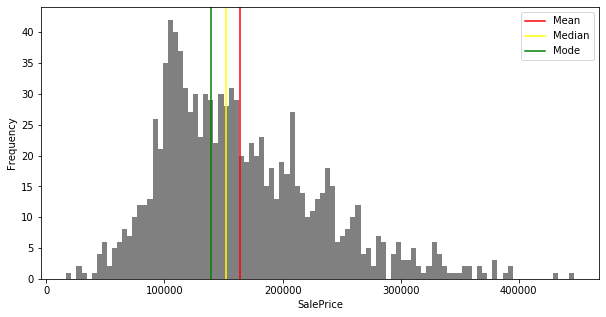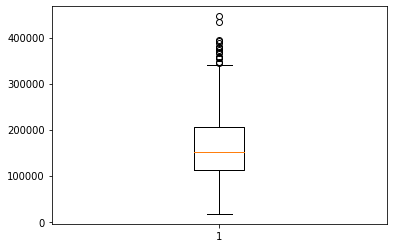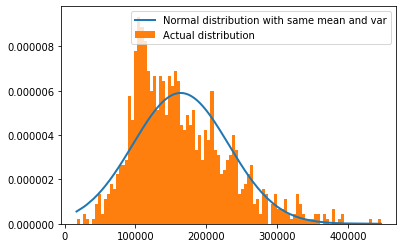### Basic Statistics for data science¶

Statistics is a science dealing with the collection, analysis, interpretation, and presentation of numerical data. - Websters dictionary
In any business, including in Retail, statistics are used extensively to do the following tasks:

1. Quantify different KPI's to get a realistic view of the business
2. Identify the cause-effect relationships between different factors
3. Create hypothesis tests to validate business intuition
4. Identify if an event has a significant effect on the business

The study of statistics can be organised in a variety of ways. One of the main ways is to subdivide statistics into two branches: descriptive statistics and inferential statistics. To understand the difference between descriptive and inferential statistics, definitions of population and sample are helpful.

#### Population¶

It is a collection of persons, objects, or items of interest. The population can be a widely defined category, such as “all products,” or it can be narrowly defined, such as “all products in Store 2105 Bedford Extra.” A population can be a group of people, such as “all Tesco's employees,” or it can be a set of objects, such as “All grocery sold on February 3, 2007”.
The analyst defines the population to be whatever he or she is studying. For example, if we want to study the effect of Christmas holidays on sales in the UK, the population would be the sales in all stores in the UK during the Christmas period. If the study is to predict the sales for the next year using the last three years patterns, then the population would be the sales of the last three years and the next year. Any analysis or predictions should be made within the population.

#### Sample¶

A sample is a portion of the whole and, if rightly taken, is representative of the whole. For various reasons, analysts often prefer to work with a sample of the population instead of the entire population. Because of time and money limitations, a human resources manager might take a random sample of 40 employees instead of using a census to measure company morale.

#### Inferential vs descriptive analytics¶

If a business analyst is using data gathered on a group to describe or reach conclusions about that same group, the statistics are called descriptive statistics. For example, if an analyst produces statistics (KPI's) to summarise a store's performance and uses those statistics to reach conclusions about that store only, the statistics are descriptive.

Another type of statistics is called inferential statistics. If a researcher gathers data from a sample and uses the statistics generated to reach conclusions about the population from which the sample was taken, the statistics are inferential. The data gathered from the sample are used to infer something about a larger group (population).

#### Parameters vs statistics¶

A descriptive measure of the population is called a parameter. Examples of parameters are population mean ($\mu$), population variance ($\sigma^2$), and population standard deviation ($\sigma$).
A descriptive measure of a sample is called a statistic. Examples of statistics are the sample mean ($\bar x$), sample variance ($s^2$), and sample standard deviation (s).

## Data measurement¶

There are four types of data. They are:

1. Nominal: Nominal data do not have a rank. They are data that are used to classify and categorise. Examples are employee identification number or sub-group information. An employee with number 5367 is not one greater than an employee with number 5368.
2. Ordinal: In ordinal data, the data can be ranked, but the difference between the two ranks should not have a meaning. This is also used for classifying data. For example, “Good”, “Average”, “Bad”. Good is a greater rank than average, and the average is greater than bad. But the difference between good and average does not have any meaning.
3. Interval: Interval is data in which the distances between consecutive numbers have meaning, and the data are always numerical. For interval data, zero is just another point on the scale and not the absence of the phenomenon. Example of interval data is Fahrenheit scale of temperature.
4. Ratio: Ratio data have the same properties as interval data, but ratio data have an absolute zero, and the ratio of two numbers is meaningful. Examples are height, weight, time etc.

## Measures of central tendency¶

Measures of central tendency tend to describe the middle part of the data. They are:
Mean: Mean is the average of a group of numbers $\mu = \frac{\sum x_i}{N} = \frac{x_1+x_2 + ... + x_n}{N}$
Median: Median is the middle value in an ordered array of numbers
Mode: Mode is the most frequently occurring value in a set of data

Percentiles: Percentiles are measures of central tendency that divide the data into 100 parts. There are 99 percentiles because there are 99 dividers to separate the data into 100 parts. The nth percentile is the value such that at least n percent of the data is below that value and at most (100 - n) percent is above that value. For example, 87th percentile means at least 87% of the data are below the value, and no more than 13% are above the value.

Quartiles: Quartiles are measures of central tendency that divide a group of data into four subgroups or parts. The three quartiles are denoted as Q1, Q2, and Q3. The first quartile, Q1, separates the first, or lowest, one-fourth of the data from the upper three-fourths and is equal to the 25th percentile. The second quartile, Q2, separates the second quarter of the data from the third quarter. Q2 is located at the 50th percentile and equals the median of the data. The third quartile, Q3, divides the first three-quarters of the data from the last quarter and is equal to the value of the 75th percentile.

## Measures of variability¶

Measures of variability are used to describe the spread or the dispersion of a set of data. They are:
Range: The range is the difference between the largest value of a data set and the smallest value of a set.
Interquartile Range: The interquartile range is the range of values between the first and third quartile. Essentially, it is the range of the middle 50% of the data and is determined by computing the value of Q3 - Q1.Mean Absolute Deviation: The mean absolute deviation (MAD) is the average of the absolute values of the deviations around the mean for a set of numbers. $MAD = \frac{\sum |x_i-\mu|}{N}$
Variance: The variance is the average of the squared deviations about the arithmetic mean for a set of numbers. The population variance is denoted by $\sigma^2$. $\sigma^2 = \frac{\sum(x_i-\mu)^2}{N}$
Standard Deviation: The standard deviation is the square root of the variance. The population standard deviation is denoted by $\sigma$. $\sigma = \sqrt(\frac{\sum(x_i-\mu)^2}{N})$

## Measures of shape¶

Measures of shape are tools that can be used to describe the shape of a distribution of data. They are:
Skewness: Skewness is when a distribution is asymmetrical or lacks symmetry. Coefficient of skewness is defined as $S_k = \frac{3(\mu-M_d)}{\sigma}$ where $M_d$ is the median.Kurtosis: Kurtosis is defined as the amount of peakedness in the distribution. There are three types of kurtosis, Loptokurtic, Mesokurtic, Platykurtic distributions.Not all measures can be used on all data types. The below table explains what measures can be used on what kind of data types:

Measure Nominal Ordinal Interval Ratio
Mean No No Yes Yes
Median No Yes Yes Yes
Mode Yes Yes Yes Yes
Percentiles No No Yes Yes
Quartiles No No Yes Yes
Range No Yes Yes Yes
Interquartile Range No No Yes Yes
MAD No No Yes Yes
Variance No No Yes Yes
Std.dev No No Yes Yes
Skewness No No Yes Yes
Kurtosis No No Yes YesThe above figure shows the relationships of the usage potential among the four levels of data measurement. The concentric squares denote that each higher level of data can be analysed by any of the techniques used on lower levels of data but, in addition, can be used in other statistical techniques.

In :
import numpy as np
import pandas as pd
import matplotlib.pyplot as plt

path="Data/house_prices.csv"
df = pd.read_csv(path)
df.head()

Out:
Price Living Area Bathrooms Bedrooms Lot Size Age Fireplace
0 142212 1982 1.0 3 2.00 133 0
1 134865 1676 1.5 3 0.38 14 1
2 118007 1694 2.0 3 0.96 15 1
3 138297 1800 1.0 2 0.48 49 1
4 129470 2088 1.0 3 1.84 29 1
In :
saleprice = df['Price']

mean=saleprice.mean()
median=saleprice.median()
mode=saleprice.mode()

print('Mean: ',mean,'\nMedian: ',median,'\nMode: ',mode)
plt.figure(figsize=(10,5))
plt.hist(saleprice,bins=100,color='grey')
plt.axvline(mean,color='red',label='Mean')
plt.axvline(median,color='yellow',label='Median')
plt.axvline(mode,color='green',label='Mode')
plt.xlabel('SalePrice')
plt.ylabel('Frequency')
plt.legend()
plt.show()

Mean:  163862.12511938874
Median:  151917.0
Mode:  139079In :
#minimum value of salePrice
df['Price'].min()

Out:
16858
In :
#maximum value of salePrice
df['Price'].max()

Out:
446436
In :
#Range
df['Price'].max()-df['Price'].min()

Out:
429578
In :
#variance
df['Price'].var()

Out:
4576733423.870562
In :
#standard deviation
from math import sqrt
sqrt(df['Price'].var())

Out:
67651.55891678005
In :
#50th percentile i.e median(q2)
df['Price'].quantile(0.5)

Out:
151917.0
In :
#75th percentile
q3 = df['Price'].quantile(0.75)
q3

Out:
205235.0
In :
#25th percentile
q1 = df['Price'].quantile(0.25)
q1

Out:
112014.0
In :
#interquartile range
IQR = q3  - q1
IQR

Out:
93221.0
In :
plt.boxplot(df['Price'])
plt.show()In :
#skewness
df['Price'].skew()

Out:
0.876159910810612
In :
#kutosis
df['Price'].kurt()

Out:
0.7598074495519183
In :
import scipy.stats as stats
#convert pandas DataFrame object to numpy array and sort
h = np.asarray(df['Price'])
h = sorted(h)

#use the scipy stats module to fit a normal distirbution with same mean and standard deviation
fit = stats.norm.pdf(h, np.mean(h), np.std(h))

#plot both series on the histogram
plt.plot(h,fit,'-',linewidth = 2,label="Normal distribution with same mean and var")
plt.hist(h,normed=True,bins = 100,label="Actual distribution")
plt.legend()
plt.show()

C:\Users\IN22915367\AppData\Local\Continuum\anaconda3\lib\site-packages\ipykernel_launcher.py:11: MatplotlibDeprecationWarning:
The 'normed' kwarg was deprecated in Matplotlib 2.1 and will be removed in 3.1. Use 'density' instead.
# This is added back by InteractiveShellApp.init_path()